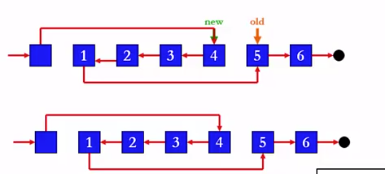Scott's world.

# Rerversing Linked List

Word count: 1.2kReading time: 5 min
2019/07/04 Share### Rerversing Linked List

02-线性结构3 Reversing Linked List (25 分)

Given a constant K and a singly linked list L, you are supposed to reverse the links of every K elements on L. For example, given L being 1→2→3→4→5→6, if K=3, then you must output 3→2→1→6→5→4; if K=4, you must output 4→3→2→1→5→6.

Input Specification:

Each input file contains one test case. For each case, the first line contains the address of the first node, a positive N (≤105) which is the total number of nodes, and a positive K (≤N) which is the length of the sublist to be reversed. The address of a node is a 5-digit nonnegative integer, and NULL is represented by -1.

Then N lines follow, each describes a node in the format:

where `Address` is the position of the node, `Data` is an integer, and `Next` is the position of the next node.

Output Specification:

For each case, output the resulting ordered linked list. Each node occupies a line, and is printed in the same format as in the input.

Sample Input:

Sample Output:

#### 思路

——————————————-更新———————————————